# 简单的基于项目的协同过滤算法

## 技术详述

#### 简介

• 其中第一种是考虑用户之间的相似性，先根据被推荐的用户，寻找相似的用户，找到一个相似用户后，预测被推荐用户对相似用户喜欢的物品的评分，然后再寻找相似的用户，直到满足终止条件为止。然后这些物品中评分最高的一批就是我们要推荐给被推荐的用户的物品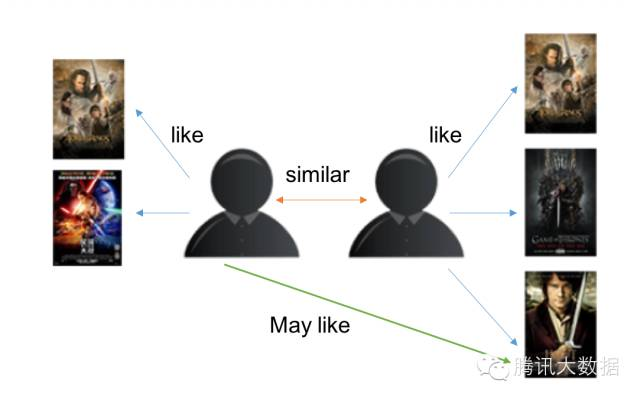• 第二种与第一种十分相似，不同点在于，不是先寻找相似用户，再预测物品的评分，而是直接寻找相似的物品，然后预测物品的评分，评分高的一批就是推荐的物品。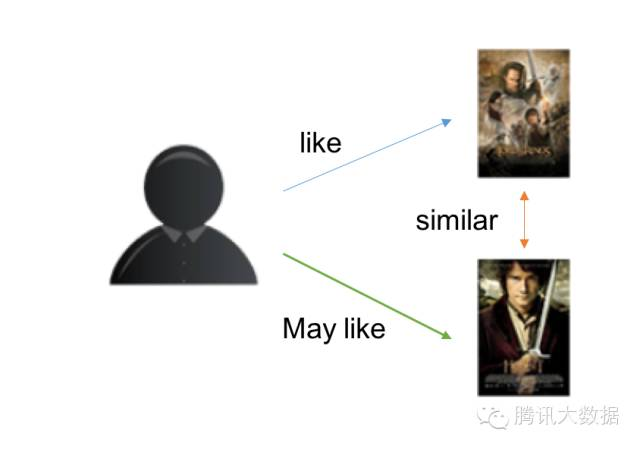• 基于模型的协同过滤作为目前最主流的协同过滤类型，其相关算法可以写一本书了，当然我们这里主要是对其思想做有一个归类概括。我们的问题是这样的m个物品，m个用户的数据，只有部分用户和部分数据之间是有评分数据的，其它部分评分是空白，此时我们要用已有的部分稀疏数据来预测那些空白的物品和数据之间的评分关系，找到最高评分的物品推荐给用户。

#### 内容

##### 公式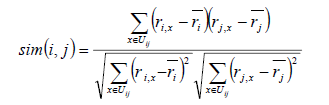I(ij)是代表用户i和用户j共同评价过的物品, R(i,x)代表用户i对物品x的评分, R(i)头上有一杠的代表用户i所有评分的平均分, 之所以要减去平均分是因为有的用户打分严有的松, 归一化用户打分避免相互影响。该公式其实就是数学中的Pearson相关系数，对于评分数据不规范时，皮尔逊相关度评价也能够给出不错的结果。

##### 步骤

• 收集用户偏好
• 找到相似的物品
• 计算推荐
###### 1. 收集用户偏好

• 减噪
用户行为数据是用户在使用应用过程中产生的，它可能存在大量的噪音和用户的误操作，我们可以通过经典的数据挖掘算法过滤掉行为数据中的噪音，这样可以是我们的分析更加精确。
在本项目中，由于技术能力不够，没有对用户进行减噪
• 归一化
如前面讲到的，在计算用户对物品的喜好程度时，可能需要对不同的行为数据进行加权。但可以想象，不同行为的数据取值可能相差很大，比如，用户的查看数据必然比购买数据大的多，如何将各个行为的数据统一在一个相同的取值范围中，从而使得加权求和得到的总体喜好更加精确，就需要我们进行归一化处理。
本项目采用了最简单的归一化处理，就是将各类数据除以此类中的最大值，以保证归一化后的数据取值在 [0,1] 范围中。

###### 2. 找到相似的物品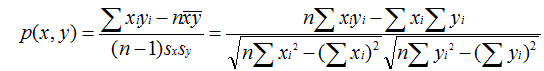Java实现代码:

/**
* 计算皮尔逊相关系数
* @param mapX 向量A
* @param mapY 向量B
* @return 两个回答的Pearson相关系数，值在[-1,1]间
*/
public static double caculatePearson(Map<BigInteger, Double> mapX, Map<BigInteger, Double> mapY) {
// (sum(X*Y) - (sum(X)*sum(Y)/N))/sqr((sum(pow(X))-(pow(sum(X))/N)) * ((sum(pow(Y))-(pow(sum(Y))/N))))
double sumXY = 0d;
double sumX = 0d;
double sumY = 0d;
double sumPowX = 0d;
double sumPowY = 0d;
Set<BigInteger> setItem = new HashSet<>();
for (Map.Entry<BigInteger, Double> entry : mapX.entrySet()) {
}
for (Map.Entry<BigInteger, Double> entry : mapY.entrySet()) {
}
for (BigInteger bookId : setItem) {
Double x = mapX.get(bookId);
if (x == null) {
x = 0d;
}
Double y = mapY.get(bookId);
if (y == null) {
y = 0d;
}
sumXY += x * y;
sumX += x;
sumY += y;
sumPowX += Math.pow(x, 2);
sumPowY += Math.pow(y, 2);
}
int n = setItem.size();
double pearson = (sumXY - sumX * sumY / n) / Math.sqrt((sumPowX - Math.pow(sumX, 2) / n) * (sumPowY - Math.pow(sumY, 2) / n));
return pearson;
}


###### 3. 计算推荐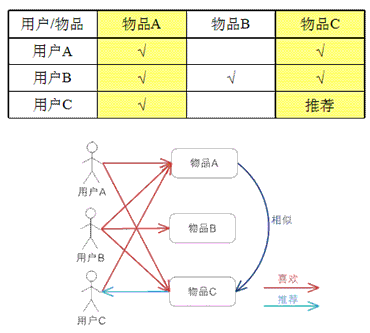## 参考文献

posted @ 2020-06-15 22:24  一年生  阅读(936)  评论(1编辑  收藏  举报